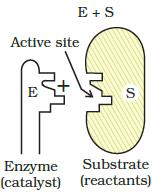Courses

# Test: Surface Chemistry- 1

## 25 Questions MCQ Test Chemistry for JEE | Test: Surface Chemistry- 1

Description
This mock test of Test: Surface Chemistry- 1 for JEE helps you for every JEE entrance exam. This contains 25 Multiple Choice Questions for JEE Test: Surface Chemistry- 1 (mcq) to study with solutions a complete question bank. The solved questions answers in this Test: Surface Chemistry- 1 quiz give you a good mix of easy questions and tough questions. JEE students definitely take this Test: Surface Chemistry- 1 exercise for a better result in the exam. You can find other Test: Surface Chemistry- 1 extra questions, long questions & short questions for JEE on EduRev as well by searching above.
QUESTION: 1

### In physical adsorption increases when:

Solution:

adsorption is inversely proportional to the temperature. This is true for physical adsorption. In chemical adsorption due to the high energy of activation, the extent of adsorption increases initially and decreases as the temperature increases further.

QUESTION: 2

### Which among the following is a property of physisorption?

Solution:

In physisorption no chemical reaction, only physical interactions hence, not specific.

QUESTION: 3

### Powdered substances are more effective adsorbents than their crystalline forms because:

Solution:

Greater the surface area, greater will be the area available for adsorption.

QUESTION: 4

Which among the following is true for chemisorptions?

Solution:

As chemical reaction takes place in chemisorption, it cant be reversed.

QUESTION: 5

In physisorption, adsorption is generally

Solution:

Physiorption includes physical forces of attraction, hence, multilayered is possible.

QUESTION: 6

Chromatography is based on the principle of

Solution:

Physical force of attraction between watt man filter paper (stationary phase) and adsorbent (mobile phase).

QUESTION: 7

The extent of adsorption increases with the ______.

Solution:

More the surface area, more is available space for adsorption.

QUESTION: 8

A biochemical catalyst is:

Solution:

Enzymes are biological catalyst, they catalize different chemical reactions in body.

QUESTION: 9

The number of phases in colloidal system are:

Solution:
• Colloidal system is a mixture of two substances, one of which, called the dispersed phase (or colloid), is uniformly distributed in a finely divided state through the second substance, called the dispersion medium (or dispersing medium).
• So, here are 2 phases.
QUESTION: 10

Which among the following substances is an example of multimolecular colloids?

Solution:

Multimolecular colloids are formed when a large number of atoms or molecules of a substance aggregate together to form speices having size in colloidal range. sulphur sol contains a thousand of S8 molecules.

QUESTION: 11

Which among the following is not the method of colloidal purification?

Solution:

Electrical Disintegration is not a method of colloidal purification.

QUESTION: 12

Tyndall effect in colloidal solution is due to:

Solution:

Tyndall effect is defined as scattering of light by colloidal particles.

QUESTION: 13

Adsorption isobar is a curve showing variation of adsorption ______.

Solution:

Isobar means when pressure is constant.

QUESTION: 14

Which step of enzyme catalysis mechanism is shown in figure?Solution:

Substrate bind to specific site of enzyme known as active site to form a enzyme substrate complex.

QUESTION: 15

Which of the following statement is true about Langmuir’s adsorption?

Solution:

Langmuir isotherm assume monolayer formation only.

QUESTION: 16

When adsorption of oxalic acid is carried out on activated charcoal, then activated charcoal is known as

Solution:

Surface on which adsorption occurs is known as adsorbent.

QUESTION: 17

Which of the following metal solution cannot be prepared by Bredig’s arc method?

Solution:

Potassium due to very high reactivity, canot be used here.

QUESTION: 18

The average molecular mass of colloidal can be determined by

Solution:

Average molecular mass of colloidal solution is determined by Osmotic Pressure measurement.

QUESTION: 19

Which of the following processes does not involve a catalyst?

Solution:

Thermite process doesn’t require a catalyst.

QUESTION: 20

Which type of a property is the Brownian movement of colloidal solution?

Solution:

Movement is always a mechanical property.

QUESTION: 21

Ammonia is adsorbed by

Solution:

Charcoal act as an adsorbent.

QUESTION: 22

Which among the following is adsorbed greatly by activated charcoal?

Solution:

SOdue to high polarity and surface area is adsorbed maximum.

QUESTION: 23

Which among the following is an example of sorption?

Solution:

When chalk is dipped in ink, absorption and adsorption both takes place which is called sorption.

QUESTION: 24

The adsorbent used in decolouration of vinegar and sugar solution is

Solution:

It adsorbs the colour in solutions.

QUESTION: 25

The adsorbent used to adsorb the dye particles in the dying industry is

Solution:

Alum has pores on it which adsorbs the color of dye and makes the solution colorless.
Hence D is correct.